#2018 iT 邦幫忙鐵人賽DAY 28
0
Modern Web

## 【DAY28】transform的matrix屬性

`transform`的一個屬性：`matrix`(矩陣)

githubpage有範例可以玩

## 一.何為矩陣？`transform`的其他四個屬性分布是`translate`(位移)、`scale`(縮放)、`rotate`(旋轉)、`skew`(傾斜)

## 二.`matrix`表示法

`transform: matrix(a,b,c,d,e,f);`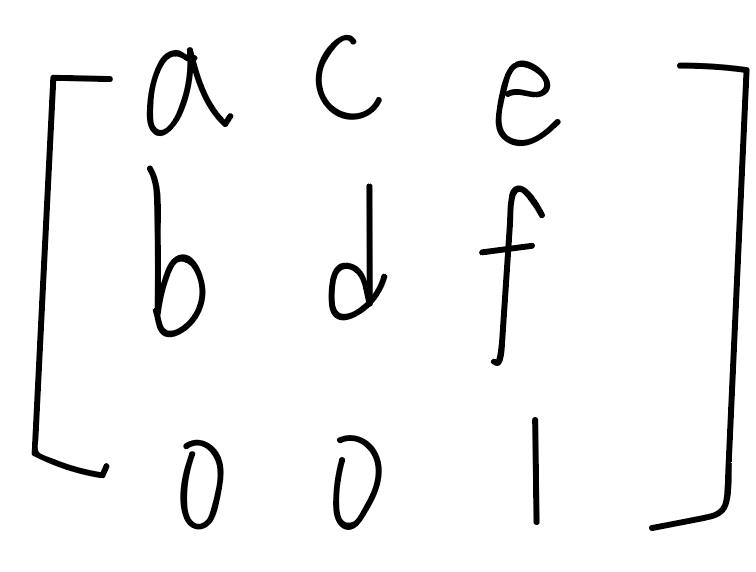## 三.何為矩陣的運算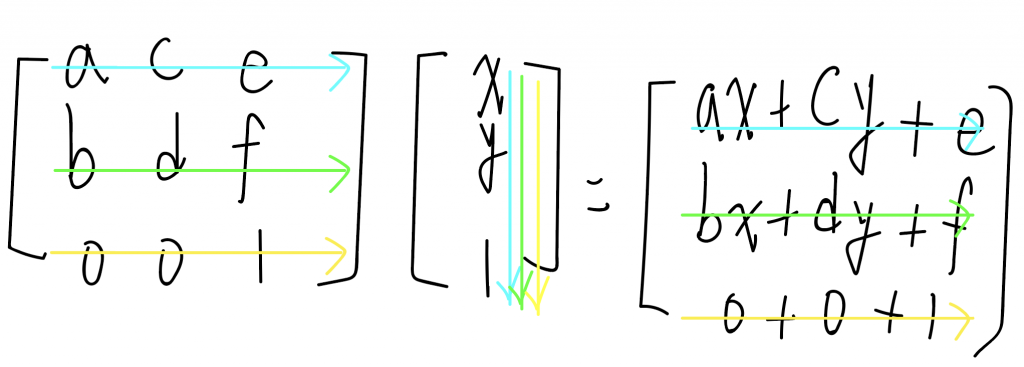「這裏交給我！你快去找多佛朗明哥！」

「你就由我來對付，魯夫他可是要成為海賊王的男人」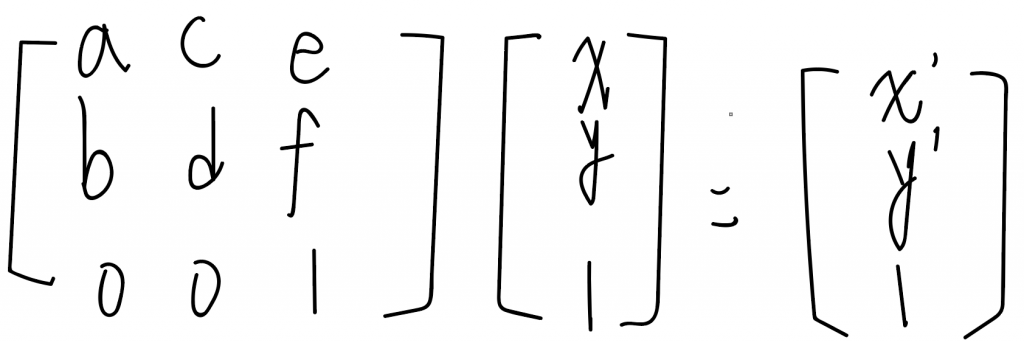x'=ax+cy+e=0+0+20=20; y'=bx+dy+f=0+0+20=20

martix VS translate

## 縮放(scale)

（中文：後來的x座標是原來x座標的兩倍；後來的y座標是原來y座標的兩倍。）

martix VS scale

## 旋轉(rotate)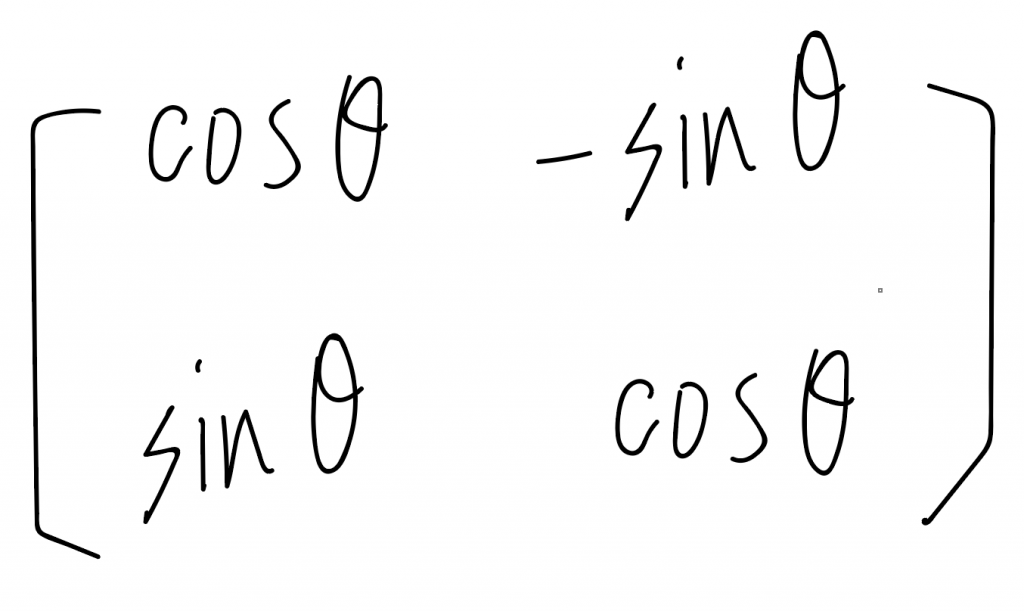`matrix(cosθ,sinθ,-sinθ,cosθ,0,0)`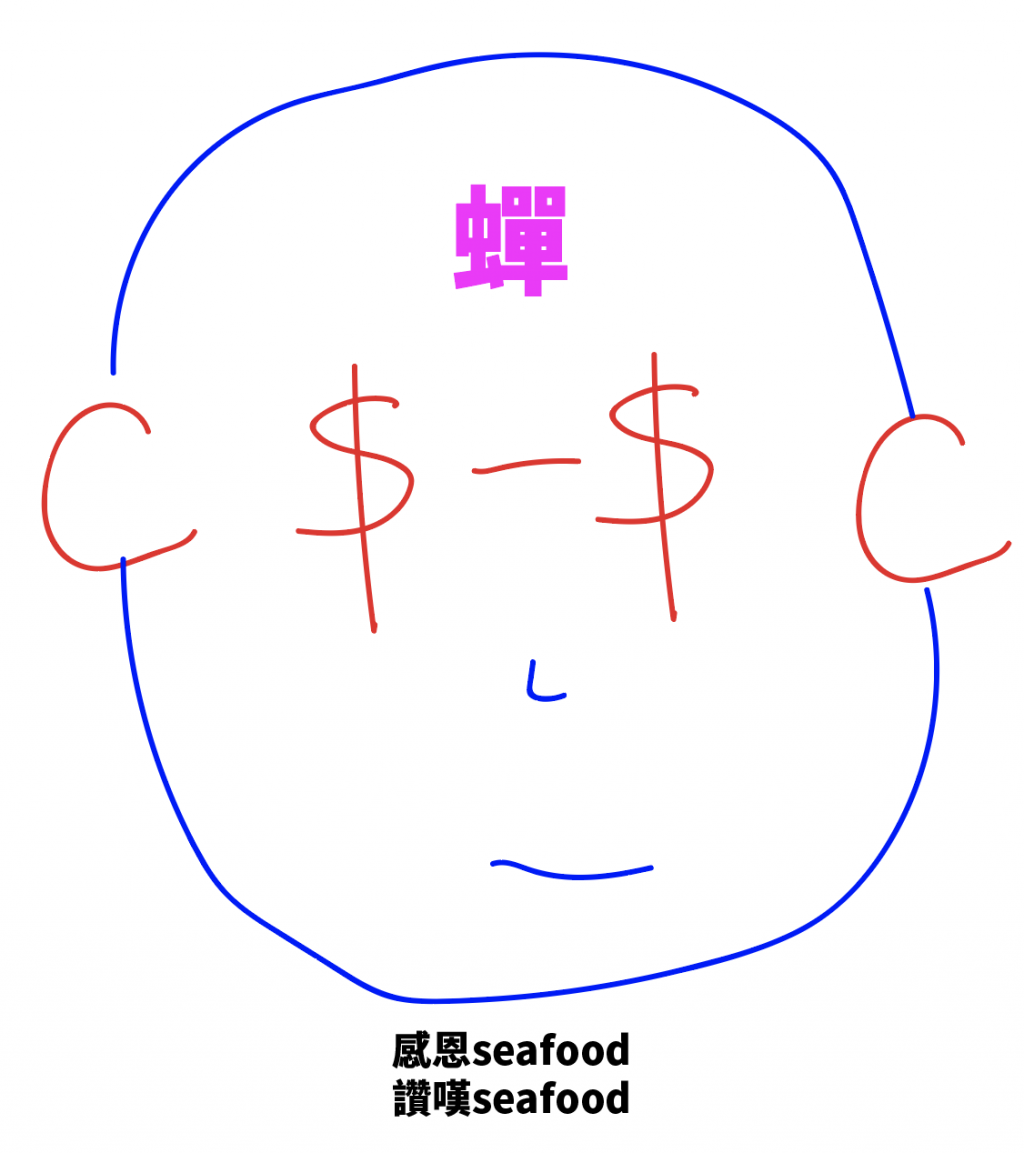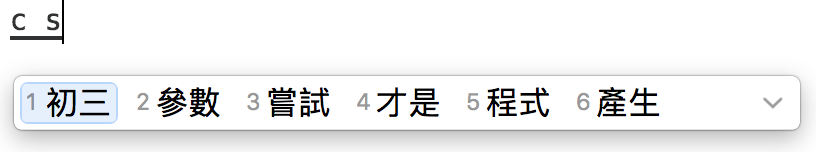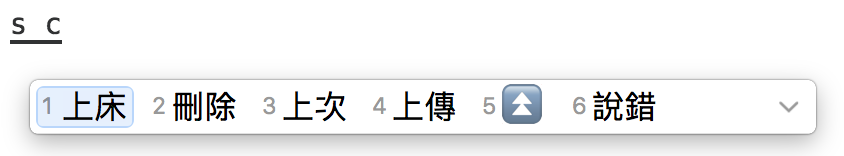`transform:rotate(30deg);``transform: matrix(0.866025,0.500000,-0.500000,0.866025,0,0);`

martix VS rotate

## 傾斜(skew)

tanθ高中學的不多，真的無法深入介紹了。

martix VS skew

`matrix`做什麼呢？